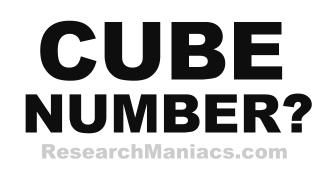Is 1 a cube number?

Cube Question: Is 1 a cube number?

For 1 to be a cube number the following equation must be true:

n3 = 1 where n is a whole number.

In other words, if n*n*n = 1, then 1 is a cube number.

If you set n=1, you get 1*1*1 which equals 1. Therefore, we can conclude that 1 is a cube number.

Answer: Is 1 a cube number? = YES

 Cube Number? Check other numbers to see if they are cube. List of Cube Numbers Here is a list of cube numbers.